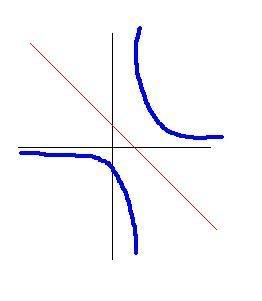# Determining the Line of Symmetry of a Reciprocal Equation.

Problem: How do you determine the line of symmetry of a reciprocal equation?

Solution:

For example, I'll graph the reciprocal function Y=1/(x+2)^Just a quick sketch

And the equation of the line of symmetry is simply -(x+2), which can be seen here:^Also a quick sketch

By adding a negative in front of any reciprocal equation, you have the line of symmetry. That much I figured out.

The Real Problem: Why does this happen? I mean, how can I explain it in plain english?